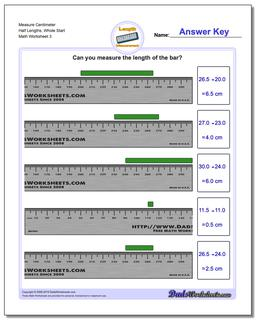# Math Worksheets: Metric Measurement: Metric Measurement: Measure Centimeter Half Lengths, Whole Start (Third Worksheet)## Measure Centimeter Half Lengths, Whole Start (Third Worksheet)

PropertyValue
DescriptionMeasure Centimeter Half Lengths, Whole Start: Measurement worksheets for measuring the length of an object placed at arbitrary whole centimeter positions on a metric ruler. These problems provide great applied practice for subtracting measurements. (Third Worksheet)
Resource TypeWorksheet